## NCERT Solutions for Class 9 Maths Chapter 9 Quadrilaterals Ex 9.1

NCERT Solutions for Class 9 Maths Chapter 9 Quadrilaterals Ex 9.1 are part of NCERT Solutions for Class 9 Maths. Here we have given NCERT Solutions for Class 9 Maths Chapter 9 Quadrilaterals Ex 9.1.

 Board CBSE Textbook NCERT Class Class 9 Subject Maths Chapter Chapter 9 Chapter Name Quadrilaterals Exercise Ex 9.1 Number of Questions Solved 12 Category NCERT Solutions

## NCERT Solutions for Class 9 Maths Chapter 9 Quadrilaterals Ex 9.1

Question 1.
The angles of quadrilateral are in the ratio 3 : 5 : 9 : 13. Find all the angles of the quadrilateral.
Solution:
Given: the ratio of the angles of quadrilateral are 3 : 5 : 9 : 13.
Let the angles of the quadrilateral are 3x, 5x, 9x and 13x.
We know that, sum of angles of a quadrilateral = 360°
∴ 3x + 5x+ 9x+13x = 360°
⇒ 30x = 360° ⇒ x = $$\frac { { 360 }^{ \circ } }{ 30 }$$ = 12°
∴ Angles of the quadrilateral are 3x = 3 x 12 = 36°
5x = 5 x 12 = 60°
9x= 9×12 = 108°
and 13x = 13×12 = 156°

Question 2.
If the diagonals of a parallelogram are equal, then show that it is a rectangle.
Solution:
Let given parallelogram is ABCD whose diagonals AC and BD are equal, i.e., AC = BD.
Now, we have to prove that ABCD is a rectangle.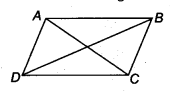Proof: In ∆ ABC and ∆ DCB, we have
AB = CD (Opposite sides of parallelogram)
BC = CB (Common in both triangles)
and AC = BD (Given)
∴ ∆ABC ≅ ∆DCB (By SSS rule)
∴ ∆ABC = ∠DCB …(i)
(Corresponding Part of Congruent Triangle)
But DC || AB and transversal CB intersect them.
∴ ∠ABC+ ∠DCB = 180°
(∵ Both are interior angles on the same side of the transversal)
⇒ ∠ABC + ∠ABC = 180° [From Eq. (i)]
⇒ 2 ∠ ABC = 180°
⇒ ∠ABC = 90°= ∠DCB
Thus, ABCD is a parallelogram and one of angles is 90°.
Hence, ABCD is a rectangle.
Hence proved.

Question 3.
Show that if the diagonals of a quadrilateral bisect each other at right angles, then it is a rhombus.
Solution:
Given: a quadrilateral ABCD whose diagonals AC and BD bisect each other at right angles.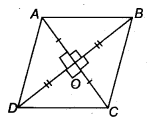i.e, OA = OC and OB = OD
and ∠AOD = ∠AOB = ∠COD = ∠BOC = 90°
To prove: ABCD is a rhombus.
Proof: In ∆OAB and ∆ODC, we have
OA = OC and OB = OD (Given)
∠AOB = ∠COD (Vertically opposite angles)
∴ ∆OAB ≅ ∆OCD (By SAS rule)
∴ AB = CD ,..(i)
(Corresponding part of congruent triangles)
Again, in ∆OAD and ∆OBC, we have
OA = OC and OD = OB (Given)
and ∠AOD = ∠BOC (Vertically opposite angle)
∴ A OAD = A OCB (By SAS rule)
∴ AD = BC …(ii) (Corresponding part of congruent triangles)
Similarly, we can prove that
CD = BC …(iii)
Hence, from Eqs. (i), (ii) and (iii), we get
AB = BC = AD = CD
Hence, ABCD is a rhombus.
Hence proved.

Question 4.
Show that the diagonals of a square are equal and bisect each other at right angles.
Solution:
Given: A square ABCD whose diagonals AC and BD intersect at O.i. e, . AC = BD, OD = OB, OA = OC and AC ⊥ BD
Proof: In A ABC and A BAD, we have
AB = BA (Common)
BC= AD (Sides of a square)
∠ABC = ∠BAD = 90°
∴ ∆ABC ≅ ∆BAD (By SAS rule)
AC = BD
(Corresponding Parts of Congruent Triangle)
∴ ∆OAB and ∆OCD
AB = DC (Sides of a square)
∠ OAB = ∠DCO
(∵ AB || CD and transversal AC intersect)
and ∠ OBA = ∠BDC
(∵AB|| CD and transversal BD intersect)
∴ ∆OAB ≅ ∆OCD
OA = OC and OB = OD
(Corresponding Parts of Congruent Triangle)
Now, in ∆AOB and ∆AOD, we have
OB = OD (Prove in above)
AB = AD (Sides of a square)
AO = OA (Common)
∴ ∆ AOB = ∆AOD (By SSS)
∴ ∠AOB = ∠AOD (By CPCT)
But ∠AOB + ∠AOD-180° (Linear pair)
∴ ∠AOB = ∠AOD = 90°
Thus, AO ⊥ BDi.e.,AC ⊥ BD.
Hence, AC = BD, OA = OC, OB = OD and AC ⊥ BD
Hence proved.

Question 5.
Show that if the diagonals of a quadrilateral are equal and bisect each other at right angles, then it is a square.
Solution:
Given: A quadrilateral ABCD in which AC = BD and AC ⊥ BD such that OA = OC and OB = OD. So, ABCD is a parallelogram.To prove: ABCD is a square.
Proof: Let AC and BD intersect at a point O.
In ∆ABO and ∆ADO, we have
BO = OD (Given)
AO = OA (Common)
∠AOB = ∠AOD = 90° (Given)
∴ ∆ABO ≅ ∆ADO (By SAS)
∴ AB = AD (By CPCT)
and AD = BC (Opposite sides of parallelogram)
∴ AB = BC = DC = AD …(i)
Again, in ∆ABC and ∆BAD, we have
AB = BA (Common)
AC = BD (Given)
BC= AD [From Eq. (i)]
∴ ∆ABC = ∆BAD (By SSS)
∠ABC+ ∠ BAD =180° …(ii)
But
∠ABC = ∠ BAD = 180° (Sum of interior angles of a parallelogram)
∴ ∠ABC = ∠BAD = 90° [From Eq. (ii)]
Thus, AB = BC = CD = DA and ∠A = 90°
∴ ABCD is a square.
Hence proved.

Question 6.
Diagonal AC of a parallelogram ABCD bisects ∠A (see figure). Show that
(i) it bisects ∠C also,
(ii) ABCD is a rhombus.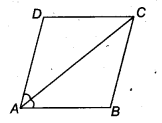Solution:
Given: diagonal AC of a parallelogram ABCD bisects ∠Ai.e., ∠DCA = ∠ BAC = $$\frac { 1 }{ 2 }$$ ∠BAD ….(i)
Here, AB || CD and AC is transversal.
∴ ∠DCA = ∠CAB (Pair of alternate angle)…(ii)
and ∠BCA = ∠ DAC (Pair of alternate angle).. .(iii)
From Eqs. (i), (ii) and (iii), we get
∠DAC = BCA = ∠ BAC = ∠DCA
Now, ∠BCD = ∠BCA + ∠DCA
= ∠DAC + ∠CAB
∴ Diagonal AC also bisects ∠C.
Again, in ∆OAD and ∆ OCD, we have
OA = OC (∵ Diagonals bisect each other)
OD = OD (Common)
∴ ∠AOD = ∠ COD = 90°
∴ ∆ OAD ≅ ∆ OCD (By SAS)
∴ AD = CD (By CPCT)
Now AB = CD and AD = BC (Opposite sides of parallelogram)
∴ AB = CD = AD = BC
Hence, ABCD is a rhombus.
Hence proved.

Question 7.
ABCD is a rhombus. Show that diagonal AC bisects ∠Aas well as ∠C and diagonal BD bisects ∠B as well AS ∠D.
Solution:
Given: ABCD is a rhombus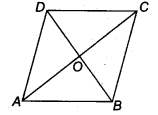∴ AD = AB = BC = CD ….(i)
To prove:
(i) Diagonal AC bisect ∠A as well as ∠C.
(ii) Diagonal BD bisects ∠B as well as ∠D.
Proof:
(i) Let AC and BD are the diagonals of rhombus ABCD.
In ∆ABC and ∆ADC,
CD = BC [From Eq,(i)]
and AC = CA (Common)
∴ ∆ABC ≅ ∆ADC ( By SSS rule)
∴ ∠DAC = ∠BAC (By CPCT)
and ∠DCA = ∠ BCA
Also, ∠DAC = ∠DCA
and ∠BAC = ∠ BCA
This shows that AC bisect ∠ A as well as ∠C.
(ii) Again, in ∆BDCand ∆BDA,
AB = BC
BD = BD (Common)
∴ ∆ BDC ≅ ∆ BDA (SSS rule)
∴ ∠BDA = ∠BDC
and ∠DBA = ∠DBC
Also, ∠BDA = ∠DBA
and ∠BDC = ∠DBC
This shows that BD bisect ∠B as well as ∠D.

Question 8.
ABCD is a rectangle in which diagonal AC bisects ∠A as well as ∠C. Show that
(i) ABCD is a square
(ii) diagonal BD bisects ∠ B as well as ∠D.
Solution:
Given: ABCD is a rectangle.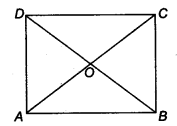∴ AB = CD and BC= AD …(i)
To Prove (i) ABCD is a square.
i.e., AB = BC = CD = DA
(ii) Dioagonal BDbisects ∠B as well as ∠D.
Proof (i) In ∆ADC and ∆ABC, we have
Since, AB || DC and AC transversal intersect
∠DAC = ∠BAC
∠DCA = ∠BCA
and AC = CA (Common)
∴ ∆ADC ≅ ∆ ABC (By ASA rule)
AD = AB (By CPCT)
and CD = BC …(ii)
Hence, from Eqs. (i) and (ii), we get
AB = BC- AD = CD
∴ ABCD is a square.
(ii) In ∆AOB and ∆COB, we have
AB = BC (Side od square)
BO = OB (Common)
OA = OC
(∵ Diagonal of square bisect each other)
∴ ∆AOB ≅ ∆COB
∴ ∠OBA = ∠OBC
This shows that BO or BD bisect ∠B.
Similarly, in ∆ AOD and ∆ COD, we have
AD = CD (Side od square)
OD = DO (Common)
and OA = OC
(∵ Diagonal of square bisect each other)
∴ ∆ AOD ≅ ∆ COD (By ASA rule)
∴ ∠ADO = ∠CDO
This shows that DO or DB bisect ∠D.
Hence proved.

Question 9.
In parallelogram ABCD, two points P and Q are taken on diagonal BD such that DP = BQ (see figure). Show that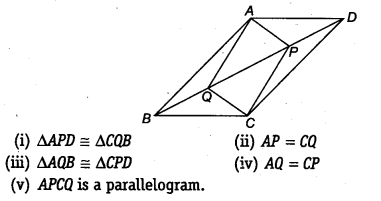Solution:
Given: ABCD is a parallelogram and P and Q are lie on BD such that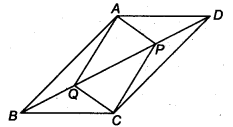DP = PQ ….(i)
(i) We have to show,
∆APD ≅ ∆CQB
Now, in ∆APD and ∆CQB, we have
DP = BQ (Given)
(Opposite sides are equal in parallelogram)
∵ AD || BC and BD is a transversal.
∴ ∠ADP = ∠QBC (Alternate interior angle)
∴ ∆APD ≅ ∆CQB (By SAS)
(ii) Since, ∆APD ≅ ∆CQB
∴ AP = CQ
(iii) Here, we have to show, ∆AQB ≅ ∆CPD
Now, in ∆AQB and ∆CPD, we have
BQ = DP (Given)
AB = CD (Opposite sides of parallelogram)
∵ AB || CD and BD is a transversal.
∴ ∠ABQ = ∠CDP (Alternate interior angle)
∴ ∆AQB s ∆ CPD
(iv) Since, ∆AQB ≅ ∆CPD
∴ AQ = CP
(v) Now, in ∆APQ and ∆PCQ, we have
AQ = CP [From part (iv)]
AP = CQ [From part (ii)]
PQ = QP (Common)
∴ ∆APQ = ∆PCQ (By SSS)
∴ ∠APQ = ∠PQC
and ∠AQP = ∠CPQ (Vertically opposite)
Now, these equal angles form a pair of alternate angle when line segment AP and QC are intersected by a transversal PQ.
∴ AP || CQ and AQ || CP
Now, both pairs of opposite sides of quadrilateral APCQ are parallel.
Hence, APCQ is a parallelogram.
Hence proved.

Question 10.
ABCD is a parallelogram and AP and CQ are perpendiculars from vertices A and C on diagonal BD (see figure). Show that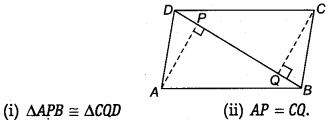Solution:
Given: ABCD is a parallelogram and AP and CQ are perpendicular from vertices A and C on diagonal BD.
∵ AB || CD and BD is a transversal.
∴ ∠CDB = ∠DBA …(i)
(i) Now, in ∆ APB and ∆ CQD, we have
CD = AB (Sides of parallelogram)
∠CQD = ∠APB = 90° (Given)
∠CDQ = ∠ABP [From Eq. (i)]
∴ ∆APB ≅ ∆CQD (By ASA rule)
(ii) ∵ ∆APB ≅ ∆CQU (By CPCT)
∴ AP = CQ
Hence proved.

Question 11.
In ∆ABC and ∆DEF, AB = DE, AB || DE, BC – EF and BC || EF. Vertices A, B and C are joined to vertices D, E and F, respectively (see figure).
Show that
(i) quadrilateral ABED is a parallelogram
(ii) quadrilateral BEFC is a parallelogram(iii) AD || CF and AD = CF
(iv) quadrilateral ACFD is a parallelogram
(v) AC = DF
(vi) ∆ABC ≅ ∆DEF
Solution:
Given: in ∆ABC and ∆DEF,
AB = DE, AB || DE
and BC = EF,BC||EF

(i)
Now, in quadrilateral ABED,
AB = DE and AB || DE (Given)
⇒ ABED is a parallelogram.
(∵ A pair of opposite sides is equal and parallel)

(ii)
BC = EF and BC || EF.
⇒ BEFC is a parallelogram.
(∵ A pair of opposite sides is equal and parallel)

(iii)
Since, ABED is a parallelogram.
∴ AD || BE and AD = BE …(i)
Also, BEFC in a parallelogram.
∴ CF || BE and CF = BE …(ii)
From Eqs. (i) and (ii), we get

(iv)
In quadrilateral ACFD, we have
AD || CF and AD = CF [From part (iii)]
⇒ ACFD is a parallelogram.

(v)
Since, ACFD is a parallelogram.
∴ AC = DF and AC || DF

(vi)
Now, in ∆ABC and ∆DEF,
AB = DE (Given)
BC = EE (Given)
and AC = DF [From part (v)]
∴ ∆ABC ≅ ∆DEF (By SSS rule)

Question 12.
ABCD is a trape∠ium in which AB || CD and AD = BC (see figure). Show that
(i )∠A=∠B
(ii )∠C=∠D
(iii) ∆ABC ≅ ∆BAD
(iv) diagonal AC = diagonal BD[Hint: Extend AB and draw a line through C parallel to DA intersecting AB produced at E].
Solution:
Given: ABCD is a trapezium.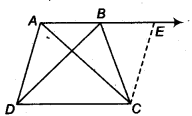AB || CD and AD = BC
Now, extend AB and draw a line through C parallel to DA intersecting AB produced at E.
Now, ADCE is a parallelogram.
∴ AD || CE and AD = CE
But AD = BC
∴ AD = BC = CE

(i)
We know that, ∠A + ∠E = 180°
(∵ Interior angles on the same side of the transversal AE)
⇒ ∠E = 180° – ∠A
Since, BC = EC
∴ ∠E = ∠CBE = 180° – ∠A
Also, ∠ ABC =180° – ∠CBE (∵ ABE is straight line)
= 180°- 180°+ ∠A
⇒ ∠B = ∠A …(i)

(ii)
Now ∠A+ ∠D = 180°
(∵ Interior angles on the same side of the transversal AD)
⇒ ∠D=180°-∠A
⇒ ∠D = 180° -∠B [From Eq. (i)]…(ii)
Also, ∠C+ ∠B = 180°
(∵ Interior angles on the same side of the transversal BC)
⇒ ∠C = 180°-∠B …..(iii)
From Eqs. (ii) and (iii), we get
∠C = ∠D

(iii)
Now, in ∆ABC and ∆ BAD, we have
AB = BA (Common)
AD = BC (Given)
∠A=∠B [From Eq. (i)]
∴ ∆ABC ≅ ∆ BAD (By SAS)

(iv)
Since, ∆ABC ≅ ∆BAD
∴ AC = BD
Hence proved.
We hope the NCERT Solutions for Class 9 Maths Chapter 9 Quadrilaterals Ex 9.1 help you. If you have any query regarding NCERT Solutions for Class 9 Maths Chapter 9 Quadrilaterals Ex 9.1, drop a comment below and we will get back to you at the earliest.